• 互换数是什么
2022-04-06 19:38:39
public class study1<num2, num1, a> {
public static void main(String[] args) {

int num1=10,num2=20;
//交换num1和num2,方法1,比较推荐方法一
int a = num1;
num1 = num2;
num2 = a ;
System.out.println(num1);
System.out.println(num2);
System.out.println(a);
System.out.println("--------------------------------------");
//方法2,可以不需要开辟单独的空间
//缺点是容易超出数据表示范围，有局限性
num1=10;
num2=20;
num1 = num1+num2;
num2=num1-num2;
num1=num1-num2;
System.out.println("num1="+num1);
System.out.println("num2="+num2);
System.out.println("--------------------------------------");
//方式三，使用位运算符号
num1=10;
num2=20;
num1=num1^num2;
num2=num1^num2;
num1=num1^num2;
System.out.println("num1="+num1);
System.out.println("num2="+num2);

}

}java
更多相关内容
• * 方法一：实现两个交换 **/ public class Exchange1 { public static void main(String[] args) { /** * 随机生成两个固定序列的0-100之间的整数， * 其中101表示生成的范围区间在：[0-101) */ Random ...
• Excel日期与数字格式的互换.rar,本例内容包括：1、文本格式转为日期；2、将日期转为文本。Excel应用
• ## C语言数字互换位置

千次阅读 2020-07-01 21:22:12
#include<stdio.h> int main (void) { int cu=1,jiangyou=2,kong; printf("%d %d\n",cu,jiangyou); kong = jiangyou ; jiangyou = cu; cu =kong; printf("%d %d\n",cu,jiangyou);... }
#include<stdio.h>
int main (void)
{
int cu=1,jiangyou=2,kong;
printf("%d %d\n",cu,jiangyou);
kong = jiangyou ;
jiangyou = cu;
cu =kong;
printf("%d %d\n",cu,jiangyou);
return 0;
}

展开全文• 主要介绍了不使用中间变量，C++实现a、b的值互相转换操作，感兴趣的小伙伴们可以参考一下
• 主要介绍了php实现子字符串位置相互对调互换的方法,可实现简单字符串中两个子字符串互换的功能,涉及php字符串运算与插入、替换等操作的相关技巧,需要的朋友可以参考下
• ## 实现两个数互换的八种方法

千次阅读 多人点赞 2020-03-08 17:39:35
目录基本数据类型借助第三个变量不借助第三个变量异或引用数据类型 基本数据类型 ... * 方法一：实现两个交换 **/ public class Exchange1 { public static void main(String[] args) { /** ...

# 基本数据类型

## 借助第三个变量

/**
* @author guqueyue
* @Date 2020/3/6
* 方法一：实现两个数交换
**/
public class Exchange1 {
public static void main(String[] args) {
/**
* 随机生成两个固定序列的0-100之间的整数，
* 其中101表示生成的数范围区间在：[0-101)
*/
Random random = new Random(47);
int a = random.nextInt(101);
int b = random.nextInt(101);
System.out.println("交换前：a = " + a + ", b = " + b);

/**
* 借助第三个变量实现第三个数互换
*/
int t = a; // t == a
a = b;     // a == b
b = t;     // b == t == a
System.out.println("交换后：a = " + a + ", b = " + b);
}
}


控制台输出如下：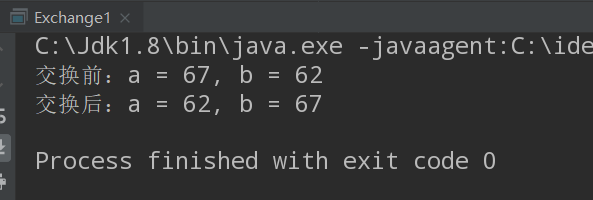如果有不明白的朋友，可以自己准备三个杯子，一个空杯代表变量t，两个杯子装上水分别代表变量a、b，然后互换一下a、b两个杯子里的水即可明白。## 不借助第三个变量

通过加减操作实现两个数互换

/**
* @author guqueyue
* @Date 2020/3/6
* 方法二：实现两个数交换
**/
public class Exchange2 {
public static void main(String[] args) {
/**
* 随机生成两个固定序列的0-100之间的整数，
* 其中101表示生成的数范围区间在：[0-101)
*/
Random random = new Random(48);
int a = random.nextInt(101);
int b = random.nextInt(101);
System.out.println("交换前：a = " + a + ", b = " + b);

a = a + b; // a == a + b
b = a - b; // b == a + b - b == a, 此时b == a
a = a - b; // a == a + b - a == b, 此时a == b
System.out.println("交换后：a = " + a + ", b = " + b);
}
}


Output: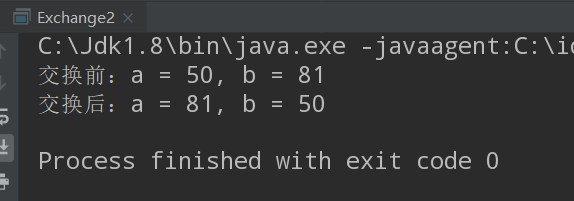通过乘除操作实现两个数互换

/**
* @author guqueyue
* @Date 2020/3/6
* 方法三：实现两个数交换
**/
public class Exchange3 {
public static void main(String[] args) {
/**
* 随机生成两个固定序列的0-100之间的整数，
* 其中101表示生成的数范围区间在：[0-101)
*/
Random random = new Random(50);
int a = random.nextInt(101);
int b = random.nextInt(101);
System.out.println("交换前：a = " + a + ", b = " + b);

a = a * b; // 此时a == a * b
b = a / b; // b == a * b / b == a, 此时b == a
a = a / b; // a == a * b / a == b, 此时a == b
System.out.println("交换后：a = " + a + ", b = " + b);
}
}


Output: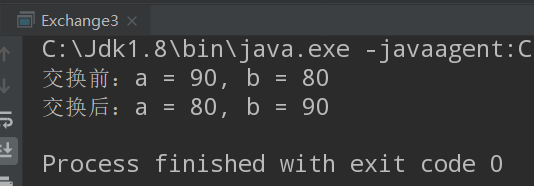### 利用赋值运算符

因为这两种方法是笔者后面补充的，所以第七种和第八种方法放在前面

利用赋值和加减来实现两个数互换

/**
* @author guqueyue
* @Date 2020/3/16
* 方法七：实现两个数互换
**/
public class Exchange7 {
public static void main(String[] args) {

/**
*  随机生成两个0-100之间的整数，
*  其中Math.random()会生成[0-1)之间任意的double类型的数
*  因此101表示生成的数范围区间在：[0-101)
*/
int a = (int) (Math.random() * 101);
int b = (int) (Math.random() * 101);
System.out.println("交换前: a = " + a + ", b = " + b);

a = b + a - (b = a); // a == b + a - a == b, a == b
System.out.println("交换后: a = " + a + ", b = " + b);
}
}


Output: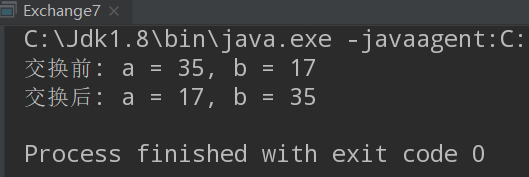利用赋值和加乘来实现两个数互换

/**
* @author guqueyue
* @Date 2020/3/16
* 方法八：实现两个数互换
**/
public class Exchange8 {
public static void main(String[] args) {

/**
*  随机生成两个0-100之间的整数，
*  其中Math.random()会生成[0-1)之间任意的double类型的数
*  因此101表示生成的数范围区间在：[0-101)
*/
int a = (int) (Math.random() * 101);
int b = (int) (Math.random() * 101);
System.out.println("交换前: a = " + a + ", b = " + b);

a = b + (b = a) * 0; // a == b + a * 0 == b, a == b
System.out.println("交换后: a = " + a + ", b = " + b);
}
}


Output: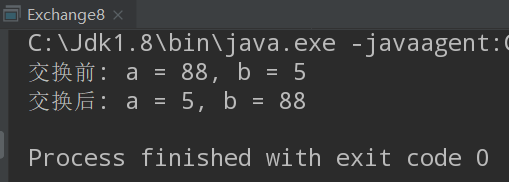### 异或

在介绍第四种方法之前，首先要跟大家介绍一下Java中的"异或"操作符(^)。
异或操作符是Java中按位操作符的一种，那么什么是按位操作符呢？

按位操作符用来操作整数基本数据类型中的单个"比特"(bit)，即二进制位。我们都知道，计算机中是采用二进制计数，而不是十进制计数。也就是说，计算机中没有我们所谓的2、3、4、5 … 100 … 1000 … ，计算机中有的只是0和1，逢二便进一。而按位操作符会对两个参数中对应的位，也就是对用二进制表示的两个参数相对应的0或1，执行布尔代数运算，并最终生成一个结果。

当然在Java中我们一般运用按位操作符很少，而我们最开始接触按位操作符，很可能是从C语言或者数字逻辑与电路。事实上，按位操作符来源于C语言面向底层的操作，这种操作经常需要直接操纵硬件，设置硬件寄存器内的二进制位。而Java的设计初衷是嵌入电视机机顶盒内，所以这种面向底层的操作被保留了下来。Java技术的三大版本之一：JavaME，Java平台微型版正是用作嵌入式开发，用来开发数字机顶盒、可视电话等电子设备。

了解了按位操作符的概念，那么接下来，我们来了解"异或"操作

如a ^ b，若a、b两个值不同，则异或结果为1；若a、b两个数相同，则异或结果为0。
大家如果要记忆的话，可以记住六字真言：同为0，异为1
或者明白或运算的朋友也可以通过字面意思来理解，若两数相(要么是0和1，要么是1和0)，则执行运算；若两数相同(同为0，或同为1)，则结果为0。

而在正式进入第四种方法之前，我们还需要了解一个变式
a ^ a = 0（同为0），b ^ 0 = b（0 ^ 0 = 0，1 ^ 0 = 1）；
所以 b ^ a ^ a = b，
也就是说一个数如果连续按位"异或"另一个数两次，那么结果等于这个数本身。

如果还是有点抽象的话，没得事，直接上代码：

/**
* @author guqueyue
* @Date 2020/3/6
* 异或操作
**/
public class Test {
public static void main(String[] args) {
/**
* 分别把结果以二进制的形式输出
*/
System.out.println("3的二进制：" + Integer.toBinaryString(3));
System.out.println("4的二进制：" + Integer.toBinaryString(4));
System.out.println("3 ^ 3 的二进制：" + Integer.toBinaryString(3 ^ 3));

System.out.print("3 ^ 0 的二进制：" + Integer.toBinaryString(3 ^ 0));
if (3 == (3 ^ 0))
System.out.println(",也就是十进制的3");

System.out.print("4 ^ 3 ^ 3 的二进制：" + Integer.toBinaryString(4 ^ 3 ^ 3));
if (4 == (4 ^ 3 ^ 3))
System.out.println(",也就是十进制的4");
}
}


Output: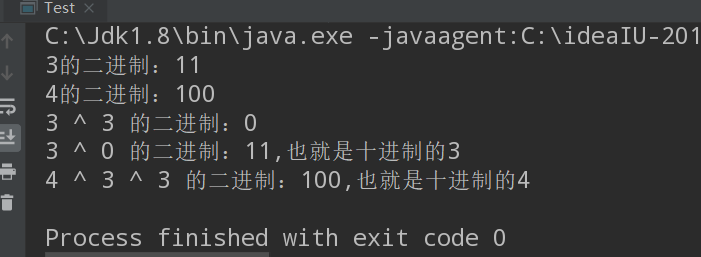好戏开场，通过异或操作实现两个数互换

/**
* @author guqueyue
* @Date 2020/3/6
* 方法四：实现两个数交换
**/
public class Exchange4 {
public static void main(String[] args) {
/**
* 随机生成两个固定序列的0-100之间的整数，
* 其中101表示生成的数范围区间在：[0-101)
*/
Random random = new Random(51);
int a = random.nextInt(101);
int b = random.nextInt(101);
System.out.println("交换前：a = " + a + ", b = " + b);

a = a ^ b; // 此时, a == a ^ b
b = a ^ b; // b == a ^ b ^ b == a, 此时b == a
a = a ^ b; // a == a ^ b ^ a == b, 此时a == b
System.out.println("交换后：a = " + a + ", b = " + b);
}
}


Output: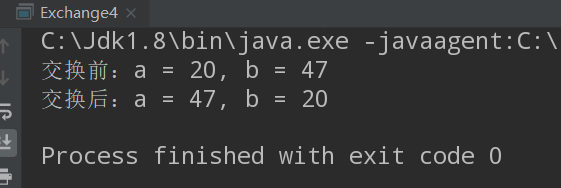# 引用数据类型

想必大家已经学了四种方法，已经对两个数互换信心满满，那么接下来，我们来看一道面试题：

/**
* @author guqueyue
* @Date 2020/3/7
**/
public class Exchange5 {
public static void main(String[] args) {
Integer a = 10;
Integer b = 20;

swop(a, b);
// 打印结果：a = 20, b = 10
System.out.println("a = " + a + ", b = " + b);
}

private static void swop(Integer a, Integer b) {
// 完成此处代码
}
}


如代码所示，完成指定位置的代码，使得程序最后的运行结果为：a = 20, b = 10

你可能觉得这还不简单，然后"刷刷刷"完成可能如以下的代码：

private static void swop(Integer a, Integer b) {
a = a ^ b;
b = a ^ b;
a = a ^ b;
}


然后再看输出结果就傻眼了: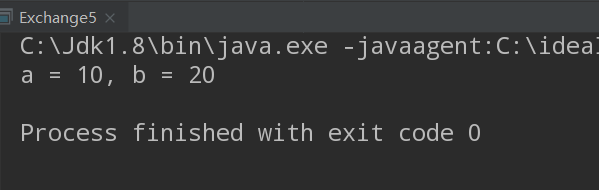纳尼，不变？这是为什么？想知道为什么的可能需要自行了解一下Java内存模型了，毕竟Java里面没有C语言的指针(小声bb)。当然，本博主以后可能也会出这方面的博客。

下面让我来揭晓正确答案吧！

private static void swop(Integer a, Integer b) throws Exception {
int x = a;
int y = b;

// 运用反射来操作Integer
Class c = Integer.class;
Field field = c.getDeclaredField("value");
// 授权访问私有
field.setAccessible(true);
// 将 a、b的值分别设置为y、x的值
field.setInt(a, y);
field.setInt(b, x);
}


当当当当，控制台输出如下：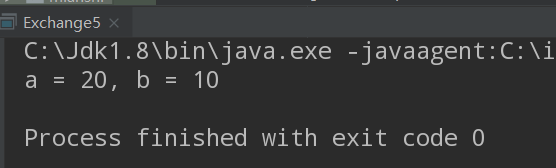至于如果有朋友想了解反射的知识，可以关注我的博客哦！毕竟反射是我当年学习JavaSE知识觉得最神奇也最喜欢的三个知识之一。

至于第六种方法嘛：

 private static void swop(Integer a, Integer b) {
System.out.println("a = " + b + ", b = " + a);
// 终止Java虚拟机的运作
System.exit(0);
}


哈哈，不要打我哦，我就皮这一下…
毕竟，题目只是说，使得运行结果为：a = 20, b = 10 即可(^_−)☆

展开全文• 展开全部swap( int x, int y)实现对x和y值的交换 ， 但从函数定义情况看，只是对形参进行了数据修改，不会影62616964757a686964616fe78988e69d8331333366306465响到实参数据。因此，你的结果不会变化 ， 仍然是a=...

展开全部

swap( int x, int y)实现对x和y值的交换 ， 但从函数定义情况看，只是对形参进行了数据修改，不会影62616964757a686964616fe78988e69d8331333366306465响到实参数据。因此，你的结果不会变化 ， 仍然是a= 1 a= 2

正确方法：

int a=1,b=0;

System.out.println("a=" + a + ";b=" + b);

int tmp;

tmp=a;

a=b;

b=tmp;

System.out.println("a=" + a + ";b=" + b);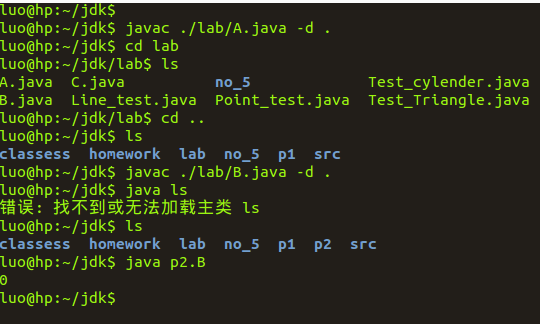扩展资料:

对于下述程序，试分析用传值、传地址、传数组方法传递参数时所得的打印结果。

PROGRAM SS(input,output);

VAR

A,B:integer;

PROCEDURE P(x,y,z:integer);

begin y:=y+1;z:=z+x;

end;

BEGIN

A:=2;b:=3;

P(A+B,A,A);

writeln (‘A=‘,A);

END

解答

(1)传值：把实参的值计算出来传给形参。

在调用过程P时，形参x=5;y=2;z=2

出过程P时，形参x=5;y=3;z=7

这并不把结果回送到主程序，所以结果为A=2

(2)传地址：实参计算出结果，把地址送形参。

设变量T=A+B(结果为5)。执行时把T、A、A的

执行过程P即为：①y↑:=y↑+1;②z↑:=z↑+x↑

所以，①为A:=A+1=3

②为A:=A+T=8。 因此，最后A=8.

(3)传数组：相当于执行 A:=2;B:=3; A:=A+1;A:=A+(A+B)

writeln(‘A=‘,A);

所以，结果为A=9。

展开全文java两个数交换
• 实现两个数互换的八种方法基本数据类型借助第三个变量package贪心;/*作者:XiangLin创建时间:2020/9/1522:48文件:XX.javaIDE:IntelliJIDEA*/importjava.util.Random;publicclassExchange1{publicstaticvoidmain...
• 输入任意五个，找出其中最小的，并和最后一个数字互换位置</p>
• 关于排列的一个简单结论证明———互换排序中任意两个，排序的逆序奇偶性改变 引言：在大学线性代数中，有关于行列式的一个常见的定理：*互换任意两行（列），行列式变号。*由行列式完全展开式可以很明显的得出...
• 要求：使用c语言互换ab两个的值 其中a，b是需要更换的两个的值，c是中间值 话不多说，直接上图说明 1.首先将a的值赋予中间值c 2.再将b的值赋予a 3.最后将中间值c的值赋予b 代码演示： #include <...
• 十六进制与双精度浮点数互换软件，经测试合格，小工具一个，方便大家开发
• 2.1（通过递归来得出一个每一位） void print(int a) { if(a>9) print(a/10); printf(“%d “,a%10); } void main() { int n; printf(“输入一个，打印它的各个位”); scanf(“%d...
• 当需要采用C语言对两个进行互换时，最先想到的是在程序中引入一个辅助变量进行实现，程序如下： 运行结果如下，能成功实现互换： 由于这种实现方式复用性不高，虽然能实现互换功能，但是实际使用时并不方便。要想...
• 写一个函数名为convert，实现将给定的3× 3的整型数组转置(行列互换)。 要求：(1)由键盘按矩阵的形式输入3× 3的整型数组。 (2)函数的原型为：void convert(int b) （3）在源程序中书写必要的注释。
• 用指针完成两个互换（整型） 实验要点 形参 无法改变 实参，可以使用指针通过调用函数完成两个互换 实验步骤 1.打开VS2010，创建新项目，选择空项目并命名，在源文件中添加新建项，选择C++文件并命名，...
• 手把手C语言入门—互换两个数字普通方式普通方式—封装一个方法普通方式—检测变量的地址在单独方法中互换... 互换两个 */ main(){ int a = 100; int b = 200; printf("互换前a=%d\n",a); printf("互换前b=...指针
• ASCII码大小写字母与数字互换公式 ASCII码值大小：数字<大写字母<小写字母 公式： 1、ASCII码大写字母转换为数字：大写字母 - ‘A’ + 1 或者 大写字母 - ‘@’ 代码如下： #include <iostream>...
• 然后再将分离出来的数字(千位、百位、十位)分别乘以10的N（N对应千、百、十可变）次方进行相加即可得到位置互换后的数字。 参考代码： #include<stdio.h> int main() { int num,gw,sw,bw,qw,result=0; ...
• 实现两个整数的互换，即输入a=x b=y 得a=y b=x 方法一：占位法 将数据单独放入一个新的变量中，进行调换。 #占位法（普通方法） print("请输入两个整数：") a = int(input("第一个整数：")) b = int(input("第二...python
•  我们都知道java中两个a,b互换，需要借助第三方变量作为临时变量来存储数据，再进行互换 在这里我提供两个不需要临时变量的方法，自己娱乐使用，仅供大家参考   int a=3,b=5; a=a+b; b=a-b; a=...位运算
• java实现数组的奇偶数互换算法需求算法思路代码实现 算法需求 定义一个数组变量，将其中的元素进行调换，奇数放到数组左边，偶数放到数组右边 算法思路 偶数放右边，奇数放左边，可以先for遍历数组，如果是奇数，...
• C语言——互换两个的整数部分 要求： 输入两个实数，互换它们的整数部分。 代码： #include<stdio.h> int main(void) { double a,b,c,d; scanf("%lf%lf",&a,&b); c=(int)b+a-(int)a;//此处的...
• 本文主要介绍了互换的概念、利率互换、货币互换互换市场等内容
• 指针程序：实现两个互换 主函数 int main(void) { int a = 3; int b = 5; swap();//调用函数实现ab的值互换 printf("a= %d,b = %d\n",a,b ); return 0; } 第一种 void swap(a,b){ int t; t = p; ...指针
• 文件内容如下： 123abc456 456def123 567adc789 789def567 要求输出： 456ABC123 123DEF456 789ADC567 567DEF789 实现代码如下： #!/bin/bash while read line do part1=`echo \$line | cut... part2shell
• 编写函数FindMax()，输入10个整数，用函数编程将其中的最大与最小数位置互换，然后输出互换后的数组。 输入 输入10个整数，相邻两项之间用一个空格隔开。 输出 输出互换后的数组，相邻两项之间用一个空格隔开。 ...
• 两个变量的值互换在面试中也会经常出现，如果可以说出多种方法，也会让面试官眼前一亮，今天小shy就给大家介绍几种方法，看看哪种更适合你。 1. 临时变量法 let a = 3, b = 5, c = b; // c: 5 b = a; // b: 3 a = c......